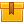# AC/DC Motor Theory Bundle

None.

## Description

This library was designed to provide training for electricians, mechanics, and others that need to learn more about AC and DC motor theory. This library consists of eleven lessons that address various aspects of AC and DC motor theory.

## Objectives

Identify the components of an AC/DC Motor. Train in the components of an AC/DC motor and explain their functions.

## ContentThis is the first lesson in the iKNOW™ AC/DC Motor Theory Library. This
lesson identifies the components of an AC motor and explains their
functions. Basic magnetic principles, sine waves, methods of increasing
magnetic flux in a conductor, and how a rotating field is created in an
AC Motor are presented.

•Introduction to AC Components and MotorsThe second lesson in the iKNOW™ AC/DC Motor Theory Library, this lesson
explains synchronous speed and how to calculate it. The lesson
demonstrates the relationship between phased current and rotor spin and
induction and its effect on a rotor. Slip and how to calculate slip
using its formula are also covered.

•ACDCMotorTheoryAdvACMotorPrincThis is the third lesson in the iKNOW™ AC/DC Motor Theory Library. This
lesson defines and explains the components and functions of various
three-phase motors. The lesson also defines torque and explains its role
in motor operation.

•Three Phase MotorsPart1This is the fourth lesson in the iKNOW™ AC/DC Motor Theory Library. This
lesson defines and explains the components and functions of externally
excited motors, starters, and variable speed drives. There is also a
review topic to reinforce the information covered in the lesson,
Three-Phase Motors – Part 1.

•ACDC Motor Theory Three Phase MotorsPt2This is the fifth lesson in the iKNOW™ AC/DC Motor Theory Library. This lesson trains the participants to distinguish single-phase motors from three-phase motors. Split-phase motors and capacitance start motors are discussed.
•Single Phase MotorsThis is the sixth lesson in the iKNOW™ AC/DC Motor Theory Library. This
lesson introduces the learner to DC Motors and their basic components.

•Introduction to DCMotorsThis is the seventh lesson in the iKNOW™ AC/DC Motor Theory Library. The lesson introduces participants to DC motor theory.

•Series Shunt DC MotorsThe eighth lesson in the iKNOW™ AC/DC Motor Theory Library, this lesson
demonstrates armature reaction, compensation, and induced voltage.

•Armature Reaction_ Compensation_ &amp; Induced VoltageThis is the ninth lesson in the iKNOW™ AC/DC Motor Theory Library.  This
lesson instructs the participant in the design of series wound, shunt
wound, and compound DC motors and how they work.

•Series Shunt DC MotorsThis is the tenth lesson in the iKNOW™ AC/DC Motor Theory Library. This
lesson instructs the student in the design of permanent magnet,
universal, and brushless DC motors and how they work.

•Permanent Magnet Universal BrushlessDCMotorsThis is the final lesson in the iKNOW™ AC/DC Motor Theory Library. This
lesson trains participants in starters, rotation direction, speed
control, and drive controls of DC motors.

•DC Motor Controls

0
0 Reviews